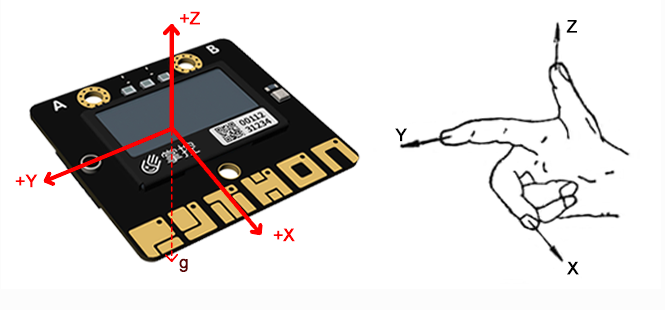# 加速度

• X - 向前和向后倾斜。
• Y - 向左和向右倾斜。
• Z - 上下翻转。``````    from mpython import *

while True:
oled.fill(0)
x1 = accelerometer.get_x()
y1 = accelerometer.get_y()
z1 = accelerometer.get_z()
oled.DispChar("加速度x:", 0, 0)
oled.DispChar(str(x1), 48, 0)
oled.DispChar("加速度y:", 0, 16)
oled.DispChar(str(y1), 48, 16)
oled.DispChar("加速度z:", 0, 32)
oled.DispChar(str(z1), 48, 32)
oled.show()``````

``    from mpython import *``

``````    x1 = accelerometer.get_x()
y1 = accelerometer.get_y()
z1 = accelerometer.get_z()``````

• 平放桌面 --(0,0,-1)
• 翻转平放桌面 --(0,0,1)
• 掌控板下板边直立与桌面 --(1,0,0)
• 掌控板左板边直立与桌面 --(0,1,0)

#### 注解：

``````发现什么规律没有？当重力加速度与加速度轴方向一致时，即等于1g的地球重力加速度。正方向为+1g，反方向为-1g。

## 水平球

``````from mpython import *    #导入mpython模块

Center_x=63           #设定中心点（原点）x的坐标
Center_y=31           #设定中心点（原点）y的坐标

while True:

x=accelerometer.get_x()         #获取X轴的加速度
y=accelerometer.get_y()         #获取Y轴的加速度

if y<=1 and y>=-1:
offsetX=int(numberMap(y,1,-1,-64,64))   #映射Y轴偏移值
if x<=1 and x>=-1:
offsetY=int(numberMap(x,1,-1,32,-32))   #映射X轴偏移值
move_x=Center_x+offsetX                 #水平球在X坐标上的移动
move_y=Center_y+offsetY                 #水平球在Y坐标上的移动

oled.circle(Center_x,Center_y,6,1)      #画中心固定圆：空心
oled.fill_circle(move_x,move_y,4,1)     #画移动的水平球：实心
oled.DispChar("%0.1f,%0.1f" %(x,y),85,0)    #显示水平球在X、Y轴的加速度值

if offsetX==0 and offsetY==0:
rgb.fill((0,10,0))          #水平球在中心位置亮绿灯，亮度为10
rgb.write()
else:
rgb.fill((0,0,0))           #水平球不在中心位置灭灯
rgb.write()
oled.show()
oled.fill(0)
````````````    if y<=1 and y>=-1:
offsetX=int(numberMap(y,1,-1,-64,64))
if x<=1 and x>=-1:
offsetY=int(numberMap(x,1,-1,32,-32))``````

#### 注解：

``numberMap(inputNum, bMin, bMax, cMin, cMax) 是映射函数，``inputNum`` 为需要映射的变量，``bMin`` 为需要映射的最小值，``bMax`` 为需要映射的最大值，``cMin`` 为映射的最小值，``cMax`` 为映射的最大值。   ``

``````    move_x=Center_x+offsetX
move_y=Center_y+offsetY ``````

``````    if offsetX==0 and offsetY==0:
rgb.fill((0,10,0))          #水平球在中心位置亮绿灯，亮度为10
rgb.write()
else:
rgb.fill((0,0,0))           #水平球不在中心位置灭灯
rgb.write()``````

## 计算掌控板倾斜的角度

``````# 通过y轴加速度求y轴与水平面倾斜角度

from mpython import*
from math import acos,degrees

while True:
y=accelerometer.get_y()
if y<=1 and y>=-1:
oled.DispChar('%.2f°' %deg_y,50,25)
oled.show()
oled.fill(0)``````

``````    from mpython import *
from math import acos,degrees``````

``    x = accelerometer.get_x()``

``    rad_x=acos(x)``

``    deg_x=90-degrees(rad_x)``

#### 注解：

``````* acos() 函数为返回反余弦弧度值。
* degrees() 函数为将弧度转换为角度。``````